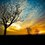The problem is that,that we are given angle BCA= 60degrees and angle BDC=45degrees, AC=2 and CD=1 and we need to find value of angle BAC without the aid of Trigonometry.Please help.

Thanks!Note by Bhargav Das
6 years, 7 months ago

This discussion board is a place to discuss our Daily Challenges and the math and science related to those challenges. Explanations are more than just a solution — they should explain the steps and thinking strategies that you used to obtain the solution. Comments should further the discussion of math and science.

When posting on Brilliant:

• Use the emojis to react to an explanation, whether you're congratulating a job well done , or just really confused .
• Ask specific questions about the challenge or the steps in somebody's explanation. Well-posed questions can add a lot to the discussion, but posting "I don't understand!" doesn't help anyone.
• Try to contribute something new to the discussion, whether it is an extension, generalization or other idea related to the challenge.

MarkdownAppears as
*italics* or _italics_ italics
**bold** or __bold__ bold
- bulleted- list
• bulleted
• list
1. numbered2. list
1. numbered
2. list
Note: you must add a full line of space before and after lists for them to show up correctly
paragraph 1paragraph 2

paragraph 1

paragraph 2

[example link](https://brilliant.org)example link
> This is a quote
This is a quote
    # I indented these lines
# 4 spaces, and now they show
# up as a code block.

print "hello world"
# I indented these lines
# 4 spaces, and now they show
# up as a code block.

print "hello world"
MathAppears as
Remember to wrap math in $$ ... $$ or $ ... $ to ensure proper formatting.
2 \times 3 $2 \times 3$
2^{34} $2^{34}$
a_{i-1} $a_{i-1}$
\frac{2}{3} $\frac{2}{3}$
\sqrt{2} $\sqrt{2}$
\sum_{i=1}^3 $\sum_{i=1}^3$
\sin \theta $\sin \theta$
\boxed{123} $\boxed{123}$

Sort by:

This can be solved without trigo, but just needs the 30-60-90 triangle. Let E be a point on BC such that CE=CD, then ACE would be a 30-60-90 triangle, so angle CEA =BEA=90. Thus angle CAE=30. Since CE=CD, and angle BCD=120, then angle CED=CDE=CAE=30, so EA=ED. Since angle BED=150 and angle BDE=15, thus angle DBE=15, and EB=EA=ED. Since angle BEA=90, we get angle BAE=45, thus angle BAC=angle BAE+angle CAE= 45+30=75.

- 6 years, 7 months ago

Sorry if I didn't follow your instructions.I think it is not easy enough to answer this using geometry only. The measure of angle BAC is 105 degrees. The proof here I will show is by using Sine Law only. The rest of the angles in triangle BCD are angle CBD = 15 degrees and angle BCD = 120 degrees.

First: By Sine Law: (sin 15) / 1 = (sin 45) / x x = (sin 45)/(sin 15) Using half-angle formula for sin 15 and the identity for sin 45, x = (sqrt 3) + 1

Furthermore: Second use of Sine Law: (sin (120 - x)) / 2 = (sin x) / ((sqrt 3) + 1) Using the sum formula for sine functions and simplifying terms, we get (sin x)/(cos x) = tan x = - (sqrt 3) - 2 Finding its inverse, we get x = -75 degrees = 105 degrees. (If you really know that x = - (sqrt 3) - 2 gives tan^(-1) of 105 degrees, then good.)

Conclusion, Angle BAC is 105 degrees. Implication: If you will notice, angle ABC is 15 degrees which implies that BC is an angle bisector that cut the segment AD into AC:AD = 2:3. I don't know if you can notice right away that BC is an angle bisector from the start. But the argument is strong that it divides the segment AD proportionally but it lacked information that BD:BA = CD:CA to determine that BC bisects the said angles..

- 6 years, 7 months ago

Thanks!

- 6 years, 7 months ago

This can be solved using coordinates

Place D, C, A on the x-axis with $D: (0, 0), C: (1, 0), A: (3, 0)$. Segment DB is contained by $y = x$ and segment CB is contained by $y = \sqrt{3}x - \sqrt{3}$. With some algebra the intersection of these two lines are $(\frac{\sqrt{3}}{\sqrt{3} - 1}, \frac{\sqrt{3}}{\sqrt{3} - 1})$. Thus, the slope of $AB$ is $-(2 + \sqrt{3})$, so $x = 75^\circ$.

On second thought, I guess this is somewhat trig because in my head I solved $\tan \theta = -(2 + \sqrt{3})$, but then again I also kind of did that for finding the slopes of the original lines. Though I'm not entirely sure.

- 6 years, 7 months ago

I never thought of using coordinates in the first place... I really thought of using theorems... By the way, how did you find that CB contained in the line y = (sqrt 3)(x - 1) as well as DB contained by y = x? Oh.. excuse me but when you solved tan(theta) = -(2 + (sqrt(3))), it should have been -75 degrees and equivalent to the measure of 105 degrees...

- 6 years, 7 months ago

$\tan 105^\circ$ is indeed $-(2 + \sqrt{3})$, however that is the exterior angle at $A$, so the interior angle is $108 - 105 = 75^\circ$.

- 6 years, 7 months ago

I should have been able to scale the triangle whether if it is acute or obtuse...

- 6 years, 7 months ago

Thanks!

- 6 years, 7 months ago

I guess it is 45 degrees

- 6 years, 7 months ago